# Reindl

This function computes the direct normal irradiance and the diffuse global irradiance from the global horizontal according to the Reindl correlation model, if no direct normal or diffuse irradiance is available. Three versions of the model are available in literature, as illustrated in Figure 6 :

1. Function of zenith angle, clearness index, air temperature, and relative humidity (blue).
2. Function of zenith angle and clearness index (red)
3. Function of clearness index only (green)

A minor modification to the published algorithm was made based on one year of hourly global and diffuse measurements at a utility-scale power plant. At very high clearness indices, the discontinuity between segments B and C of the piecewise regression model is too abrupt, leading to discontinuous irradiance profiles on even clear days.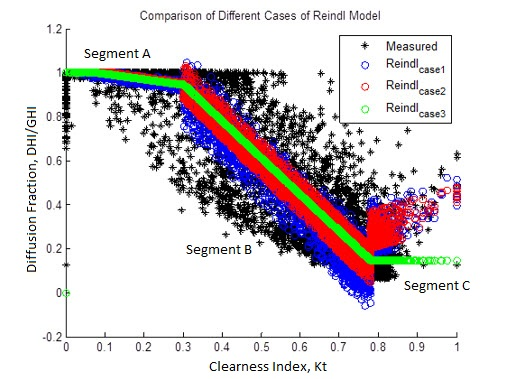### Figure 6. Reindl Diffuse-Direct Decomposition Model Compared to Measured Data

The discontinuity at Kt = 0.78 is problematic for clear sky days when there is little measured diffuse irradiance (black). An adjustment was made, as shown in Figure 7.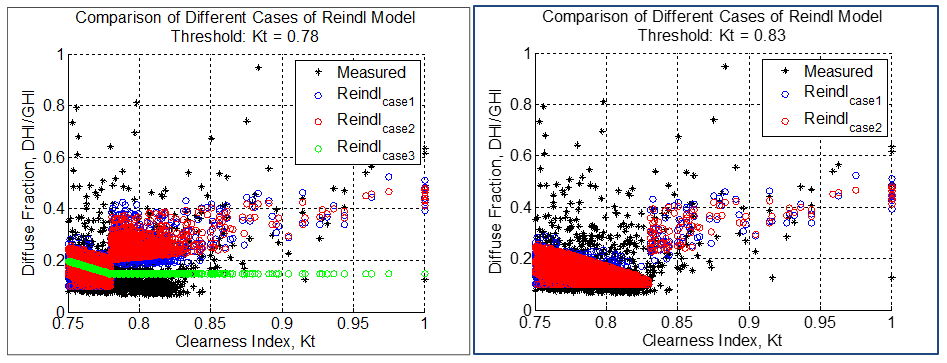### Figure 7. Modification of then Reindl Diffuse Correlation Model

This causes a large discontinuity in the errors of modeled DHI during these times. Based on this residual analysis it appears that the error in the Reindl model in sloped segment B is large up to a Kt of 0.83 – 0.84. As a result, the threshold between segments B and C was moved from 0.78 to 0.83.

## Outputs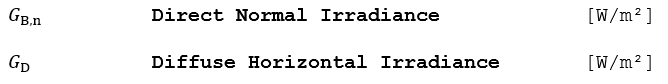## Algorithm

Only versions (1) and (2) of the algorithm (as described above) are implemented, as version (3) is not necessary since ambient temperature is required in all PlantPredict weather files. If Φ is available, version (1) is used; if Φ is missing, then PlantPredict automatically defaults to version (2).

1.) Find the piecewise correlation of the diffuse fraction if air temperature and relative humidity are known (fd, first equation), or unknown (f’d, second equation). If the diffuse fraction falls out of the allowed range, clamp the result at the boundary value. Also note that these equations use the solar zenith angle, not the solar elevation angle (as defined in the Reindl paper).

1. If 0 ≤ Kt ≤ 0.3: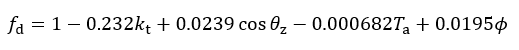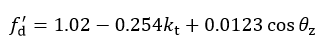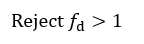1. If 0.3 < Kt < 0.83: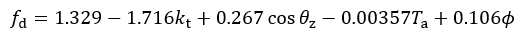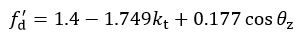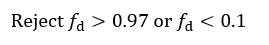1. If 0.83 ≤ Kt: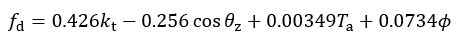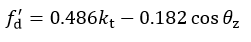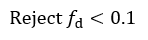2.) Compute the diffuse irradiance: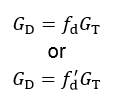3.) Compute the direct normal irradiance only if GT > GD, as follows and if θz < 89°: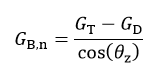Else, set G B,n to 0

## Reference

Reindl, D. T., Beckman, W. A., Duffie, J. A., Diffuse Fraction Corrections, Solar Energy Vol. 45, No. 1, pp. 1-7, 1990.